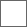• 29回复贴，共1

### 【答网友问】男友总是冷暴力逼分手，之后又说是为你好是什么心理#漠河七夕飘雪#【吧主回复】1、吵架时2、社交时3、日常思考4、压力大、焦虑时5、工作时6、日常爱好7、买车时8、其他场合》》》》》》》》》》》》》》》》》》》警戒线《《《《《《《《《《《《《《《《《《《《

(((((((((((((((((((((((((((((((((((((((((((((((((((((((((((())))))))))))))))))))))))))))))))))))))))))))))))))))))))))
))))))))))))))))))))))))))))))))))))))))))))))))))))))缓冲区))))))))))((((((((((((((((((((((((((((((((((((((((((((
((((((((((((((((((((((((((((((((((((((((((((((((((((((()))))))))))))))))))))))))))))))))))))))))))))))))))))))))))))))

~~~~~~~~~~~~~~~~~~~~~~~~~~~和平的分界线(*╹▽╹*)~~~~~~~~~~~~~~~~~~~~~~~~~~~~~…………#### 扫二维码下载贴吧客户端